# DMelt:Math/1 Functions

Limitted access. First login to DataMelt if you are a full DataMelt member. Then login to HandWiki as a user.

## Mathematical functions

Function is a relation between inputs values and a set of outputs values such that each input is related to exactly one output.

DataMelt can be used to evaluate, plot, simplify functions. There are several approaches to work with parametric or non-parametric functions using DataMelt Java API:

• User 2D/3D function plotter from DataMelt IDE (see the "Tools" menu)
• Simplify and plot functions using ether Octave or Matlab syntax. Plotting functions in such approach is a bit too simplistic and there is no good integration with Java API and data structures
• Use the full-blown DataMelt Java libraries to create parametric, non-parametric, special functions as they are provided by Java API. One can also integrate, differentiate and simplify such functions. In this case one can use the Java language, or any Java scripting languages (such as Jython or BeanShell). There is complete integration with data structures API and functions can be plotted in 2D/3D using several powerful interactive canvases.

In this section we will discuss the third option as it is the most flexible for integration with Java libraries. We will start with simple analytical functions with one variable ("x") and then will consider more complicated cases with several variables ("y", "z"). Finally, we will show how to make function of arbitrary complexity with any number of variables

# Functions with one variable

Functions can be defined using either strings or programically. First we will discuss how to show functions using strings and then we will show other, more flexible methods.

We will start this tutorial with a simple example of how to create and display a function with one and only one variable ("x"). First, let us create an object representing a function:

$\displaystyle{ f(x)=2*exp(-x*x/50)+sin(pi*x)/x }$

Such functions are represented by thejhplot.F1D Java class (1D means one-dimensional function).

from jhplot  import  *
f1 = F1D("2*exp(-x*x/50)+sin(pi*x)/x")

Here the input function is given byjava.lang.String. One can evaluate this function at a certain point, say at 10, as:

print f1.eval(10)
0.270670566473

By default, all trigonometric functions are implemented using the fast calculations with e-14 accuracy. Note, you can also use exact calculations using </javadoc>java.lang.Math</javadoc> using additional parameter:

from jhplot  import  *
HParam.setExactMath()
f1 = F1D("2*exp(-x*x/50)+sin(pi*x)/x")

The method "HParam.setExactMath()" tells that full precision of Java Math package is not required. In this case, calculations of trigonometric functions is a factor 2-3 slower. Look at the classjhplot.HParam.

The code shown below benchmark calculations of F1D function containing trigonometric functions with and without the fast math enabled.

To draw the function, one should specify limits during the function initialization (or later using the methods of the Java objectjhplot.F1D. Then one should build a canvas and use the method "draw" to plot the function. In the example below, we define the limits for this function (-2,5) and then we plot it:

from jhplot  import *
f1 = F1D("2*exp(-x*x/50)+sin(pi*x)/x", 1.0, 10.0)
c1 = HPlot("Example of  function")
c1.visible()
c1.setAutoRange()
c1.draw(f1)

Here we drop the Python invitation ">>>" since we assume that the this code snippet is executed as a file. Insert these lines to the DataMelt editor and hit the key [F8]. You will see a pop-up window with the output as shown below: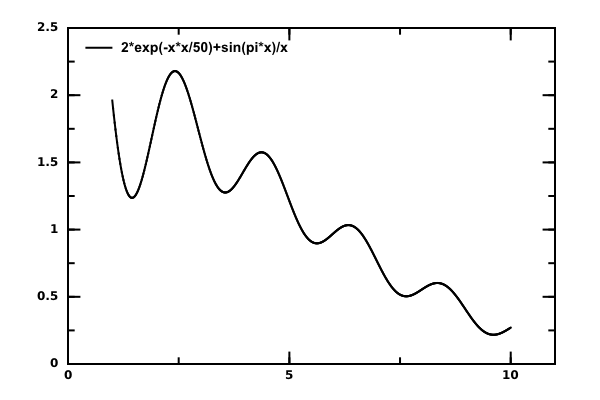To learn about the method of DataMelt or any Jython or Java objects (like f1 and c1 in the above example):

• Hit <Ctrl>+<Space> after the dot when using the Jython shell prompt (bottom part of the DataMelt IDE).
• When using the DataMelt editor, use the key [F4] (after the dot) which brings up a table with all available Java methods.
• Use Eclipse or NetBean IDE when developing the code using Java. They have their own help system.
• Read the Java API of these classes.

Here is a short description about how to define functions. The following table shows the main mathematical operations:

()     parenthesis
+      plus
-      minus
*      times
/      divide
^      raise to a power
**     raise to a power

Functions:

String Definition
abs absolute value
log(x) Natural Logarithm
log10(x) Logarithm base 10
sqrt(x) <m>sqrt{x}</m>
exp(x) Exp
ceil nearest upper integer
floor nearest lower integer
cbrt cubic root
sin(x) Sine
cos(x) Cosine
tan(x) Tangent
asin(x) ArcSine
acos(x) ArcCosine
atan(x) ArcTangent
sinh(x) Hyperbolic Sine
cosh(x) Hyperbolic Cosine
tanh(x) Hyperbolic Tangent

One can also use some predefined constants, such as pi or Pi (the pi value, 3.14..). It is possible to use Scientific notation for numbers. The number is split into a significant / mantissa (y) and exponent (x) of the form 'yEx' which is evaluated as 'y * 10^x'. Let us give the examples:

from jhplot  import *

print f1.eval(2)
print f1.eval(2)
print f1.eval(2)
print f1.eval(2)

## Parameters

Functions can have parameters. If you have parameters, you should avoid "parsing" functions, and apply parsing only after finals substitution. This example shows how to work with such functions:

f1=F1D("(3*alpha)/alpha+10+x",0) # 0 means False
f1.simplify()                    # simplify the function
print f1.getName()
f1.setPar("alpha",100)           # substitute alpha=100
f1.parse()                       # parse it for evaluation, plotting etc.
print f1.getName()
print f1.eval([1,2,3])

Please also consider the method f1.toString() that prints more information aboutjhplot.F1D.

## Function initialization

Functions can be initialized with and without ranges.

When using this syntax:

from jhplot import *
f1=F1D("x^2")

function does not have limits used for plotting and evaluation. For such definition, plot ranges are entirely determined by the plotting canvases (i.e. by the setRange() methods.

When using this syntax:

from jhplot import *
f1=F1D("x^2",-10,10) # limit to [-10,10]

The function includes limits for numeric calculation and plotting. This means you can set auto-range for plotting canvases, since the function includes the X-range in the definition, thus it will be shown in the range [-10,10], as in this example.

## Non-parametric functions

The most flexible way to draw functions is to use codding with objects and calling third-party Java libraries directly, instead of using strings with function definitions. This topic will be discussed in Section Non-parametric functions

## Symbolic manipulations

Symbolic manipulation with functions will be discussed in more details in Section Introduction. Still, one should note that symbolic manipulations can also be done with the F1D functions using Java (or Jython) coding without using any other specialized language (as Matlab or Octave). One can simplify and expand F1D functions. Functions can also be rewritten in terms of elementary functions (log, sqrt, exp). Finally, one can perform some elementary substitutions before attempting to plot a F1D function.

It is often useful to rewrite an expression in terms of elementary functions (log, exp, frac, sqrt, implicit roots), using the "elementary()" method, before simplifying it.

## Integration and differentiation

Functions can be numerically integrated. The program supports 5 methods of integration, which vary in evaluation time and accuracy. Below we will integrate the function "cos(x*x)+sin(1/x)*x^2)"

This code integrates the function between 10 and 100 using 5000 integration point (the large the number is, the more accurate the results are). The code also performs some benchmarking which gives you ideas about the accuracy of calculations:

trapezium  = 4949.64217622  time (ms)= 31.654937
gauss4     = 4949.64028115  time (ms)= 43.84014
gauss8     = 4949.64028111  time (ms)= 65.27855
richardson = 4949.64028393  time (ms)= 56.030938
simpson    = 4949.64014049  time (ms)= 17.634015

## Displaying functions

Here is a more detailed example showing how to plot several functions using different colors:

Note that we have imported the Java classjava.awt.Color. The output of this script is shown here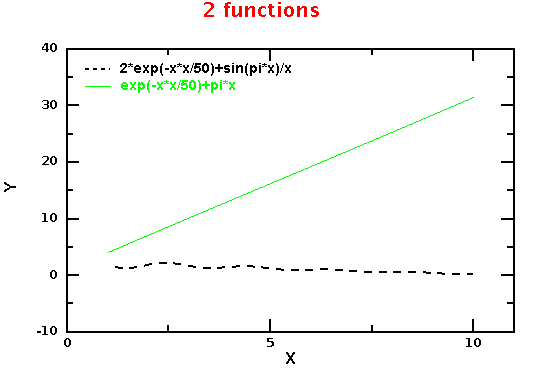You can also plot objects on different pads as shown in the Section Plotting canvases.

## Exporting functions

As any Java object, F1D functions can be saved into files. Read Input and Output for detailed discussion. In addition, one can convert a function in MathML or Java formats, or just display it as a table with values after evaluation of a function.

>>> from jhplot import F1D
>>> f=F1D("10*sin(x)")
>>> print f.toMathML()  # convert to MathML
>>> print f.toJava()    # convert to Java code
>>> f.toTable()         # show a table with X-Y values (the function should be plotted or evaluated before)

# 2D Functions

Functions in 2 dimensions can be build analogously using the Java classjhplot.F2D. The example below shows how to construct and evaluate a function 2D:

>>> from jhplot  import  *
>>> f1 = F2D("2*exp(-x*y/50)+sin(pi*x)/y")
>>> print f1.eval(10,20)
0.0366312777775

The code prints the answer: 0.270670566473.

Such functions can be displayed as will be shown later. Also, look at the Sections 2D Plots and 3D Plots

# 3D Functions

Functions in 2 dimensions can be build by analogy using the Javajhplot.F3D class. The example below shows how to construct and evaluate a function 3D:

>>> from jhplot  import  *
>>> f1 = F3D("2*exp(-x*y/50)+sin(pi*x)/z")
>>> print f1.eval(10,20,30)
0.0366312777775

The code prints the answer: 0.0366312777775.

# Converting functions to histograms

Histograms can be created from 1D and 2D functions. In the example above, we created a 2D function and then used it to generate 2D histogram. Functions can be converted to Histograms with arbitrary number of bins. This often can be used for show selected regions in different color.

Consider the example in which a function is used to create a histogram with fine bins. We use this histogram to highlight a range of our original function.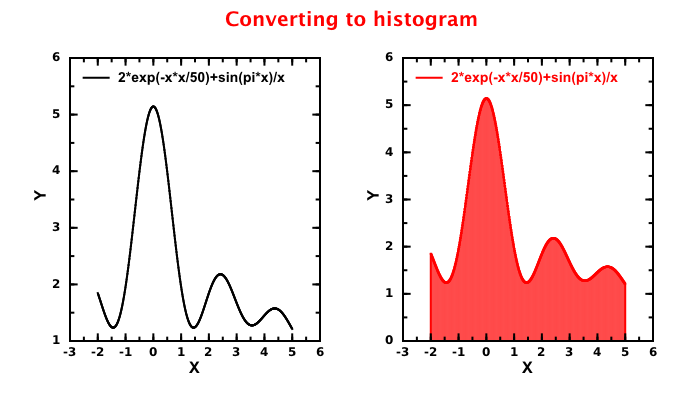Histograms can have arbitrary number of bins, but if the bin number is close to 500 (a typical evaluation step for functions), the difference between function and a histogram will be almost impossible to see.

# Plotting in 3D

F2D functions can be shown using 3D canvases. They can be overlayed and shown on a single plot. Here is a small example of showing 2 functions in 3D:

The output of this code is: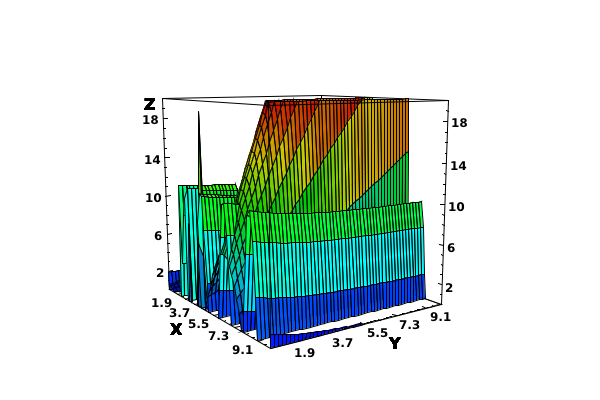One can also overlay several different objects. Let us show an example of how to show functions and histograms in 3D: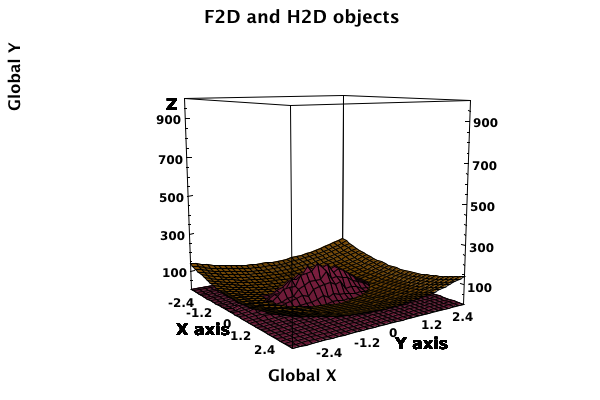The code used to generate this image is given below.

You can plot an arbitrary function in 3D usingjhplot.HJavaView by defining function programmatically, and rebuilding vertexes for surface plots. Here is how you can do it:

The output of this code is: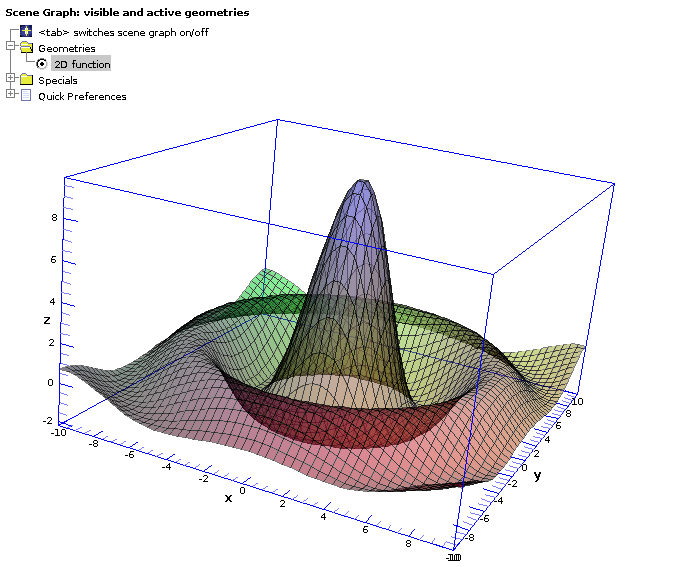You can adjust all visual characteristics of this plot using JavaView GUI.

# Multidimensional functions

So far we have learned how to build functions in 1D (F1D class), 2D (F2D class) and 3D (F3D class). In addition to these "fixed dimension" classes, there is ajhplot.FND which can be used to build a function with arbitrary parameters and variables. To build such function is very easy and similar to the above styles, only now one should be more explicit about the names of variables. As example, below we make a function with 4 variables, called xx, yy, bb, kk:

from jhplot import *
f=FND("xx*sqrt(yy)*bb*+kk",'xx,yy,bb,kk')
ans=f.eval('xx=10,yy=1,bb=0,kk=20')
print ans

Such functions can be evaluated at any variable values and plotted.

## Integration

Numeric integration of functions is discussed in section Integration. Symbolic integrations will be discussed in dedicated sections.

Let us give a small example showing how to integrate $\displaystyle{ cos(x)^3 }$ using a trapezium rule. We will integrate this function between 1 and 10 using 10k iterations.

from jhplot import F1D
f1=F1D('cos(x)^3')
print f1.integral(10000,1,10)

# Expression Builder

Multidimensional functions can also be built using the package jhplot.math.exp4j. In the example below we build a function usingjhplot.math.exp4j.ExpressionBuilder:

from java.awt import*
from jhplot  import *
from jhplot.math.exp4j import *

e=ExpressionBuilder("3 * sin(x) - 2 / (x - 2)")
e.variables("x")

ff=F1D("Test", e.build() ,10,100)
print ff.eval(10) # check at x=10

c1 = HPlot()
c1.visible(1)
c1.setAutoRange()
c1.draw(ff)

You can build an arbitrary function using Python and plot it, mixing Java and Jython libraries in one code. You can put an arbitrary complex expressions and "if" statements. In the example below we make a custom function using a Jython class and build an "ExpressionBuilder" object. then we evaluate this function for array of "X" values and plot it.

Now we can show how to create a custom function in 3D. Again, we will use a python code to do this.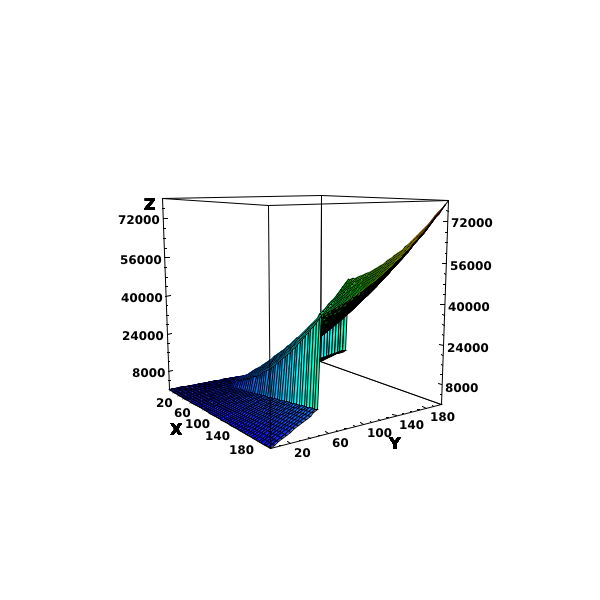# Fast Math

The implementation of trigonometric functions is a 3-4 times slower performance compare to C/C++. This is especially true for atan2(), acos(), asin() functions. By default, DMelt uses fast calculations for all F1D, F2D etc. functions. The table below shows the performance of FastMath compared to the standard Java implementation blog. The times are in mean nanoseconds.

<html>

Function Math Mean FastMath Mean Times Faster
acos 58 16 3.6
asin 57 15 3.8
atan 94 15 6.2
atan2 145 23 6.3
cbrt 112 18 6.2
ceil 10 11 0.9
cos 74 13 5.7
cosh 141 28 5.0
exp 73 16 4.6
expm1 113 17 6.6
floor 11 12 0.9
hypot 435 23 18.9
log 31 16 1.9
log10 27 13 2.1
log1p 97 15 6.5
nextAfter 11 10 1.1
nextUp 10 8 1.3
pow 101 38 2.7
round 11 10 1.1
sin 72 16 4.5
sinh 126 23 5.5
sqrt 7 15 0.5
tan 52 14 3.7
tanh 167 26 6.4
toDegrees 7 8 0.9
DMelt also include less precise methods for fast evaluation basic functions usingjhplot.math.MathUtilsd. They are faster than the functions shown in above table, but their accuracy is roughly 1E-05.